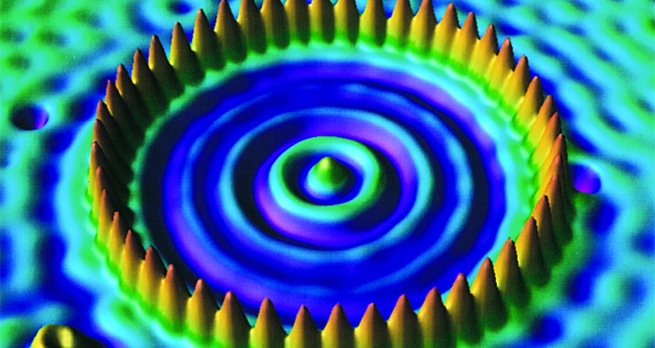Collisions and conservation laws

Start this free course now. Just create an account and sign in. Enrol and complete the course for a free statement of participation or digital badge if available.

Free course

# 2 Elastic and inelastic collisions

When starting to investigate collision problems, we usually consider situations that either start or end with a single body. The reason for this self-imposed limitation is that such problems can be solved by applying momentum conservation alone, namely the result that the total linear momentum of an isolated system is constant. The analysis of more general collisions requires the use of other principles in addition to momentum conservation. To illustrate this, we now consider a one-dimensional problem in which two colliding bodies with known masses and , and with known initial velocities and collide and then separate with final velocities and . The problem is that of finding the two unknowns and . Conservation of momentum in the -direction provides only one equation linking these two unknowns:

Equation label: (1)

which is insufficient to determine both unknowns.

In the absence of any detailed knowledge about the forces involved in the collision, the usual source of an additional relationship between and comes from some consideration of the translational kinetic energy involved. The precise form of this additional relationship depends on the nature of the collision.

Collisions may be classified by comparing the total (translational) kinetic energy of the colliding bodies before and after the collision. If there is no change in the total kinetic energy, then the collision is an elastic collision . If the kinetic energy after the collision is less than that before the collision then the collision is an inelastic collision . In some situations (e.g. where internal potential energy is released) the total kinetic energy may even increase in the collision; in which case the collision is said to be a superelastic collision .

In the simplest case, when the collision is elastic, the consequent conservation of kinetic energy means that

Equation label: (2)

This equation, together with Equation 1 will allow and to be determined provided the masses and initial velocities have been specified. We consider this situation in more detail in the next section.

Real collisions between macroscopic objects are usually inelastic but some collisions, such as those between steel ball bearings or between billiard balls, are very nearly elastic. Collisions between subatomic particles, such as electrons and/or protons, commonly are elastic. The kinetic energy which is lost in an inelastic collision appears as energy of a different form (e.g. thermal energy, sound energy, light energy, etc.), so that the total energy is conserved. Collisions in which the bodies stick together on collision and move off together afterwards, are examples of completely inelastic collisions . In these cases the maximum amount of kinetic energy, consistent with momentum conservation, is lost. (Momentum conservation usually implies that the final body or bodies must be moving and this inevitably implies that there must be some final kinetic energy; it is the remainder of the initial kinetic energy, after this final kinetic energy has been subtracted, that is lost in a completely inelastic collision.)

S217_1